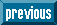## Indicators

•Cited by SciELO
•Access statistics

•Similars in SciELO

## Print version ISSN 1405-5546

#### Abstract

BARCENAS, Everardo. A Counting Logic for Trees. Comp. y Sist. [online]. 2015, vol.19, n.2, pp.407-422. ISSN 1405-5546.  http://dx.doi.org/10.13053/CyS-19-2-1999.

It has been recently shown that the fully enriched µ-calculus, an expressive modal logic, is undecidable. In the current work, we prove that this result does not longer hold when considering finite tree models. This is achieved with the introduction of an extension of the fully enriched µ-calculus for trees with numerical constraints. Contrastively with graded modalities, which restrict the occurrence of immediate successor nodes only, the logic introduced in this paper can concisely express numerical constraints on any tree region, as for instance the ancestor or descendant nodes. In order to show that the logic is in EXPTIME, we also provide a corresponding satisfiability algorithm. By succinct reductions to the logic, we identify several decidable extensions of regular tree languages with counting and interleaving operators. It is also shown that XPath extensions with counting constructs on regular path queries can be concisely captured by the logic. Finally, we show that several XML reasoning problems (XPath queries with schemas), such as emptiness and containment, can be optimally solved with the satisfiability algorithm.

Keywords : Automated reasoning; modal logics; arithmetical constraints; formal languages; XPath.

· text in English     · English (pdf )All the contents of this journal, except where otherwise noted, is licensed under a Creative Commons Attribution License